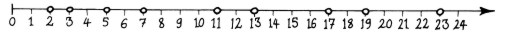A prime number is a positive whole number greater than one which is divisible only by itself and one. The first few are shown above. If the definition doesnt mean much to you, think of prime numbers as follows: If you are presented with a pile of 28 stones, you will eventually deduce that the pile can be divided into 2 equal piles of 14, 4 equal piles of 7, 7 equal piles of 4, etc. However, if one more stone is added to the pile, creating a total of 29, you can spend as long as you ike, but you will never be able to divide it into equal piles (other than the trivial 29 piles of 1 stone). In this way, we see that 29 is a prime number, whereas 28 is non-prime or composite. All composites break down uniquely into a product of prime factors: i.e. 28 = 2 × 2 × 7. Note that 2 is the only even prime – all other even numbers are divisible by 2. 1 is neither prime nor composite by convention. << previous         home         next >>